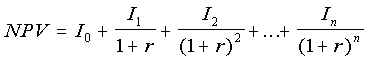How can I calculate internal rate of return (IRR)?

Internal rate of return (IRR) is a valuable calculation because it generates a projected return that can be directly compared to the company's hurdle rate. Learn how to calculate IRR in this expert tip from Tom Pisello.

What is the formula for calculating internal rate of return (IRR)?

IRR is defined as the discount rate which makes the net present value of a project equal to zero. In mathematical terms, IRR is the projected discount rate that makes the net present value (NPV) calculation equal to zero. The NPV formula is defined as:The IRR calculation is used to derive the value of r, and then given a series of net benefits (I), the equation yields zero as the NPV. The calculation is performed iteratively, where a computer program guesses at the value of r, and then continuously refines itself, until the equation yields a result at or near zero.

In practical terms, the IRR calculation examines the positive and negative cash flows from a proposed project and generates an interest rate. This rate represents the value another investment would need to generate in order to be equivalent to the cash flows of the investment being considered. For example, with NPV savings calculations, a series of net cash flows could be defined as:

Initial = I(0) = - \$100,000 Year 1 = I(1) = + \$175,000 Year 2 = I(2) = + \$175,000 Year 3 = I(3) = + \$175,000

For this set of net cash flows, the IRR calculation that yields an NPV of zero is 166%.

The IRR calculation is valuable because it generates a projected return that can be directly compared to the company's hurdle rate. Typically, the hurdle rate is the risk adjusted return a project needs to generate in order to be considered. Risk adjusted returns need to be substantially higher than those generated by safe investments to be considered equivalent. Hurdle rates across all corporate initiatives might range from as low as 15% for safe investments, to over 100% for the riskiest of projects. Due to the complexities associated with new technologies, the short lifecycles of these investments, and the process changes that accompany them, IT project returns are often highly discounted as to the reliability of achieving promised returns within the designated analysis period. IT project hurdle rates of 50% to 100% are common corporate standards.

The value of a high IRR and why hurdle rates are used can be demonstrated in capital market terms. When making a personal investment, money in a savings account is insured, but only yields a 3% to 4% interest rate, while a highly rated bond is not insured and can generate a modestly risky 5% to 6%, and an equity investment is relatively risky and returns an average gain of 10%. The equity investment needs to generate higher potential returns before you would consider taking on the risk of not having an insured investment. If the equity investment only yielded 5% to 6%, is the marginal gain worth the extra risk? This is the hurdle rate, the rate at which the investment makes sense given a specific risk profile and tolerance. A high return above the hurdle rate provides a return that exceeds the relative risk. By calculating IRR, a corporation can consider whether the projected risks of applying capital and labor resources to the project are worth the returns.

Close